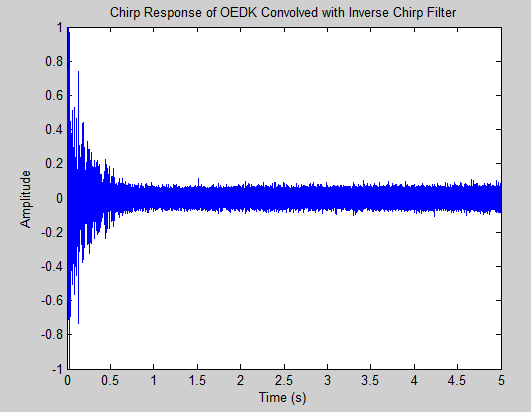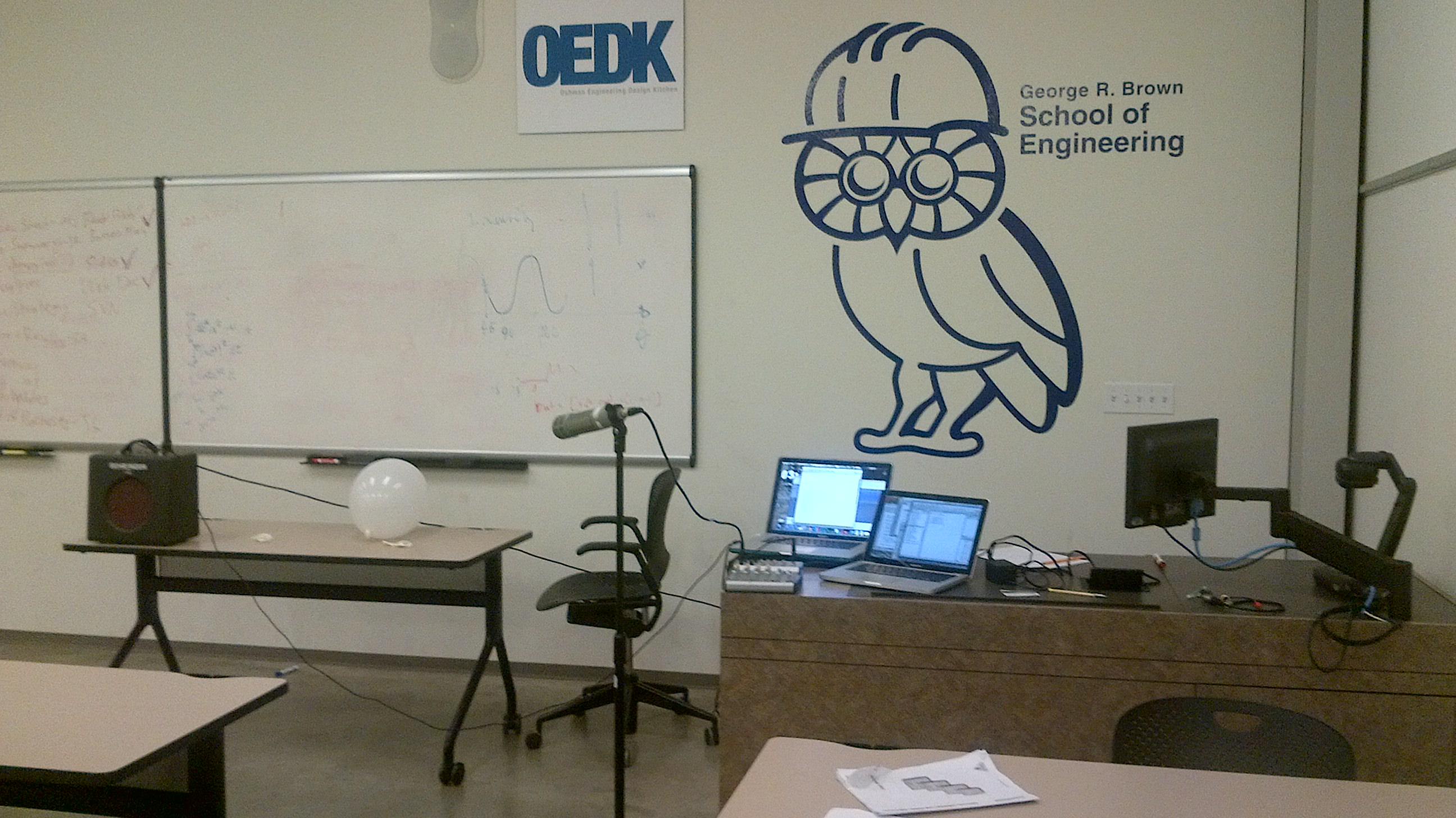# 0.2 The deconvolution method

 Page 1 / 1
The inverse filter approach to echo cancellation.

## Deconvolution

The output of a linear time-invariant (LTI) system is the convolution of the input signal with the impulse response of the system. If the classroom is modeled as an LTI system, then the output echoed signal, $y\left(t\right)$ , is the convolution of the input signal, $x\left(t\right)$ , with the room's impulse response, $h\left(t\right)$ .Block diagram of the echoic room as an LTI system with impulse response h ( t ) MathType@MTEF@5@5@+= feaagGart1ev2aqatCvAUfeBSjuyZL2yd9gzLbvyNv2CaerbuLwBLnhiov2DGi1BTfMBaeXatLxBI9gBaerbd9wDYLwzYbItLDharqqtubsr 4rNCHbGeaGqiVu0Je9sqqrpepC0xbbL8F4rqqrFfpeea0xe9Lq=Jc9vqaqpepm0xbba9pwe9Q8fs0=yqaqpepae9pg0FirpepeKkFr0xfr=x fr=xb9adbaqaaeGaciGaaiaabeqaamaabaabaaGcbaGaamiAaiaacIcacaWG0bGaaiykaaaa@3935@ where y ( t ) MathType@MTEF@5@5@+= feaagGart1ev2aqatCvAUfeBSjuyZL2yd9gzLbvyNv2CaerbuLwBLnhiov2DGi1BTfMBaeXatLxBI9gBaerbd9wDYLwzYbItLDharqqtubsr 4rNCHbGeaGqiVu0Je9sqqrpepC0xbbL8F4rqqrFfpeea0xe9Lq=Jc9vqaqpepm0xbba9pwe9Q8fs0=yqaqpepae9pg0FirpepeKkFr0xfr=x fr=xb9adbaqaaeGaciGaaiaabeqaamaabaabaaGcbaGaamyEaiaacIcacaWG0bGaaiykaaaa@3946@ is the echoed version of the input x ( t ) MathType@MTEF@5@5@+= feaagGart1ev2aqatCvAUfeBSjuyZL2yd9gzLbvyNv2CaerbuLwBLnhiov2DGi1BTfMBaeXatLxBI9gBaerbd9wDYLwzYbItLDharqqtubsr 4rNCHbGeaGqiVu0Je9sqqrpepC0xbbL8F4rqqrFfpeea0xe9Lq=Jc9vqaqpepm0xbba9pwe9Q8fs0=yqaqpepae9pg0FirpepeKkFr0xfr=x fr=xb9adbaqaaeGaciGaaiaabeqaamaabaabaaGcbaGaamiEaiaacIcacaWG0bGaaiykaaaa@3945@ .

The process of deconvolution involves designing an inverse filter ĥ(t) that is convolved with the echoed output signal to retrieve the original signal $x\left(t\right)$ . This can be done in either the time domain or frequency domain:

• Time domain: Use the deconv() method in Matlab on the echoed signal and the impulse response of the classroom in order to extract the de-echoed signal.
• Frequency domain: Take the Fast Fourier Transform (FFT) of both the impulse response of the room and the echoed signal. Point-wise divide the echoed signal by the transfer function, then take the inverse FFT of the result to extract the de-echoed signal.Inputting the echoed signal into the inverse filter yields the de-echoed signal.

However in practice, the system adds noise to input signal, meaning that if the signal-to-noise ratio is too low, the inverse filter will yield a noisy signal that poorly approximates the input.

## Finding the impulse response

A system is characterized at all frequencies by taking its impulse response . This is done by exciting a system with a Dirac delta function . The Dirac Delta Function is defined as:

$\delta \left(x\right)=\left\{\begin{array}{cc}+\infty ,& x=0\\ 0,& x\ne 0\end{array}\right\}$
$\underset{-\infty }{\overset{\infty }{\int }}\delta \left(x\right)dx=1$
The Dirac Delta Function is a distribution that is infinitely tall and infinitely narrow at 0, and the area under the Dirac Delta Function is defined to be 1.

However, it is practically impossible to excite a room with the ideal Dirac Delta function. Consequently, we used three methods to approximate the room's impulse response:

• Balloon Pop: filling a latex balloon with air and bursting it.
• Pseudo Dirac: using the dirac() function in Matlab to generate a vector of zeros with a single one in the center, then playing it using the sound() function.
• Sine-sweep Method:
1. Generate a logarithmically increasing sine signal over a desired frequency range (20 Hz to 20 KHz for this application)
2. Create an inverse chirp filter that time reverses the chirp and shifts it to become a causal signal (so that it exists in positive time). Then, divide the magnitude of the spectrum of the inverse filter by the square of the magnitude of the spectrum of the chirp signal.
The time shift inverts the phase of the chirp leading to linear phase after the convolution and the second set of operations neutralizes the squaring of the magnitude of the spectrum caused by the convolution.
• Convolve the chirp response of the room with the inverse chirp filter to get the impulse response of the room.

This logarithmically increasing sine signal can be characterized in the time domain by the following equation:

$x\left(t\right)=\mathrm{sin}\left[{\frac{T{\omega }_{1}}{\mathrm{ln}\left(\frac{{\omega }_{2}}{{\omega }_{1}}\right)}}^{\left({e}^{\frac{t}{T}\mathrm{ln}\left(\frac{{\omega }_{2}}{{\omega }_{1}}\right)}-1\right)}\right]$

where ${\omega }_{1}$ is the initial radian frequency and ${\omega }_{2}$ is the ﬁnal radian frequency of the sweep of duration $T$ .

## Sine sweepThe impulse response of the classroom is gleaned from the convolution of the room's response to the logarithmically increasing sine signal with the pre-calculated inverse chirp filter.

To measure the response, we used:

• A small guitar amplifier with a relatively flat response facing the desks
• A directional cardioid microphone 2 meters from the amplifier at a thirty degree angle
• A microphone preamplifier
• An Apple Macbook Pro Laptop using Audacity for recordingOur equipment setup in the OEDK classroom. The microphone is placed in the same location used for recording presentations.

## Problems

Several factors make this model unrealistic:

• Realistically, speakers move around and face different directions when they move. Our impulse responses are highly specific to the position of the signal's source. The information we get is based on the amplifier being stationary and facing a single direction.
• The impulse response is also time-varying. If even one person leaves, the average absorption coefficient of all the objects in the room changes and, per the Sabine equation, the reverberation time of the room also changes. People and objects are continually shifting, constantly affecting how the sound reflects around the room.

Time-varying adaptive filters are more sensible for problems such as suppressing reverberations in the OEDK classroom.

explain and give four Example hyperbolic function
_3_2_1
felecia
⅗ ⅔½
felecia
_½+⅔-¾
felecia
The denominator of a certain fraction is 9 more than the numerator. If 6 is added to both terms of the fraction, the value of the fraction becomes 2/3. Find the original fraction. 2. The sum of the least and greatest of 3 consecutive integers is 60. What are the valu
1. x + 6 2 -------------- = _ x + 9 + 6 3 x + 6 3 ----------- x -- (cross multiply) x + 15 2 3(x + 6) = 2(x + 15) 3x + 18 = 2x + 30 (-2x from both) x + 18 = 30 (-18 from both) x = 12 Test: 12 + 6 18 2 -------------- = --- = --- 12 + 9 + 6 27 3
Pawel
2. (x) + (x + 2) = 60 2x + 2 = 60 2x = 58 x = 29 29, 30, & 31
Pawel
ok
Ifeanyi
on number 2 question How did you got 2x +2
Ifeanyi
combine like terms. x + x + 2 is same as 2x + 2
Pawel
x*x=2
felecia
2+2x=
felecia
Mark and Don are planning to sell each of their marble collections at a garage sale. If Don has 1 more than 3 times the number of marbles Mark has, how many does each boy have to sell if the total number of marbles is 113?
Mark = x,. Don = 3x + 1 x + 3x + 1 = 113 4x = 112, x = 28 Mark = 28, Don = 85, 28 + 85 = 113
Pawel
how do I set up the problem?
what is a solution set?
Harshika
find the subring of gaussian integers?
Rofiqul
hello, I am happy to help!
Abdullahi
hi mam
Mark
find the value of 2x=32
divide by 2 on each side of the equal sign to solve for x
corri
X=16
Michael
Want to review on complex number 1.What are complex number 2.How to solve complex number problems.
Beyan
yes i wantt to review
Mark
use the y -intercept and slope to sketch the graph of the equation y=6x
how do we prove the quadratic formular
Darius
hello, if you have a question about Algebra 2. I may be able to help. I am an Algebra 2 Teacher
thank you help me with how to prove the quadratic equation
Seidu
may God blessed u for that. Please I want u to help me in sets.
Opoku
what is math number
4
Trista
x-2y+3z=-3 2x-y+z=7 -x+3y-z=6
can you teacch how to solve that🙏
Mark
Solve for the first variable in one of the equations, then substitute the result into the other equation. Point For: (6111,4111,−411)(6111,4111,-411) Equation Form: x=6111,y=4111,z=−411x=6111,y=4111,z=-411
Brenna
(61/11,41/11,−4/11)
Brenna
x=61/11 y=41/11 z=−4/11 x=61/11 y=41/11 z=-4/11
Brenna
Need help solving this problem (2/7)^-2
x+2y-z=7
Sidiki
what is the coefficient of -4×
-1
Shedrak
the operation * is x * y =x + y/ 1+(x × y) show if the operation is commutative if x × y is not equal to -1
A soccer field is a rectangle 130 meters wide and 110 meters long. The coach asks players to run from one corner to the other corner diagonally across. What is that distance, to the nearest tenths place.
Jeannette has $5 and$10 bills in her wallet. The number of fives is three more than six times the number of tens. Let t represent the number of tens. Write an expression for the number of fives.
What is the expressiin for seven less than four times the number of nickels
How do i figure this problem out.
how do you translate this in Algebraic Expressions
why surface tension is zero at critical temperature
Shanjida
I think if critical temperature denote high temperature then a liquid stats boils that time the water stats to evaporate so some moles of h2o to up and due to high temp the bonding break they have low density so it can be a reason
s.
Need to simplify the expresin. 3/7 (x+y)-1/7 (x-1)=
. After 3 months on a diet, Lisa had lost 12% of her original weight. She lost 21 pounds. What was Lisa's original weight?
how did you get the value of 2000N.What calculations are needed to arrive at it
Privacy Information Security Software Version 1.1a
Good
Got questions? Join the online conversation and get instant answers!

#### Get Jobilize Job Search Mobile App in your pocket Now!By JavaChamp TeamBy Richley CrapoBy Anonymous UserBy Mary MateraBy Monty HartfieldBy OpenStaxBy David CoreyBy OpenStaxByBy Sheila Lopez